``````struct VAO {
int ID;
int VBO_ID;
int EBO_ID;
// ...
}
``````

## GLSL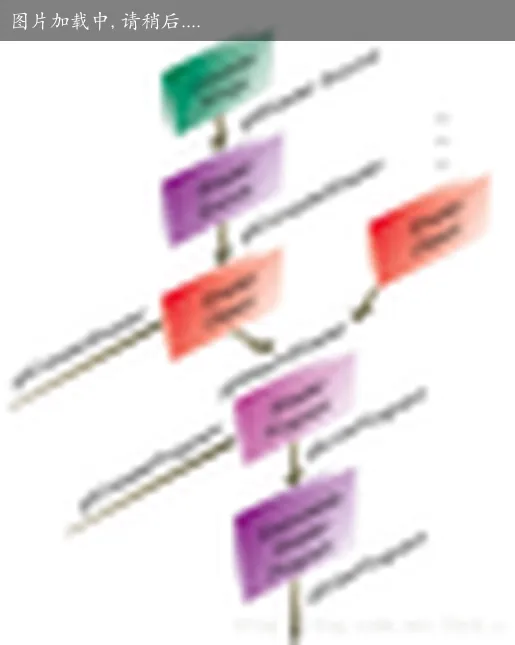### 变量限定符

• const 用于声明非可写的编译时常量变量
• attribute 用于经常更改的信息，只可以再顶点着色器中使用 [CPU -> GPU 顶点着色器]
• uniform 用于不经常更改的信息，用于顶点着色器和片元着色器 [CPU -> GPU]
• varying 用于从顶点着色器传递到片元着色器的插值信息 [GPU 顶点着色器 -> GPU 片元着色器]
``````invariant varying lowp float color; // invariant > storage > precision

void doubleSize(const in lowp float s) { // storage > parameter > precision
float s1 = s;
}
``````

``````attribute vec4 gl_Color;          // 顶点数据字段的 Diffuse 颜色
attribute vec4 gl_SecondaryColor; // 顶点数据字段的 Specular 颜色
attribute vec4 gl_Normal;         // 顶点法线
attribute vec4 gl_Vertex;         // 顶点位置
attribute vec4 gl_MultiTexCoord0; // 8 组贴图坐标
attribute vec4 gl_MultiTexCoord1;
attribute vec4 gl_MultiTexCoord2;
attribute vec4 gl_MultiTexCoord3;
attribute vec4 gl_MultiTexCoord4;
attribute vec4 gl_MultiTexCoord5;
attribute vec4 gl_MultiTexCoord6;
attribute vec4 gl_MultiTexCoord7;
attribute vec4 gl_MultiTexCoord0;
attribute vec4 gl_MultiTexCoord1;
attribute vec4 gl_FogCoord;       // 使用雾效果的参考数值
``````

``````vec4 gl_Position;                 // 用来设置顶点转换到屏幕坐标的位置，Vertex Shader 一定要去更新这个数值
float gl_PointSize;               // 是启动 PointSprite 功能时，用来设置矩形大小的数值
vec4 gl_ClipVertex;               // 如果启用了 Clip Plane 功能，gl_ClipVertex 可以放入用来与 Clip Plane 平面做测试用的位置
``````

``````varying Vec4 gl_FrontColor;           // 对正面做不同的光照计算，这两组颜色分主要颜色和次要颜色 代表的是固管的 Diffuse 值
varying vec4 gl_BackColor;            // 背面
varying vec4 gl_FrontSecondDaryColor; // 固管的 Specular 值
varying vec4 gl_BackSecondaryColor;
varying vec4 gl_TexCoord[gl_MaxTextureCoords]; // glTextCoord 是指第 0 个贴图坐标
varying vec4 gl_FogFragCoord;
``````

Fragment Sahder 除了可以从上面几个所列出的变量获得内插结果外，还可以从另外两个内置变量得到一些无法从 Vertex Shader 获得的数值。

``````vec4 gl_FragCoord;   // gl_FragCoorg.xy 代表像素在 Framebuffer 画面的位置，gl_FragCoord.z 代表这个点在做 Z Buffer 测试时所用的 Z 值
bool gl_FrontFacing; // 可用来查询目前正在画的像素是来自三角形的正面还是来自他的背面
``````

``````vec4 gl_FragColor;                   // 代表画面所要填入的颜色
vec4 gl_FragData[gl_MaxDrawBuffers]; // 用来填入画面的颜色，用在启用多个 FrameBuffer 时，调用 gl_FragData 填入画面颜色
vec4 gl_FrData                       // 填入画面颜色
vec4 gl_FragDepth;                   // 用来指定 Z Buffer 测试时所使用的 Z 值，这样就可以不通过顶点内插得到的 Z 值
``````

## GLSL 语言基础

from

### 变量

（OpenGL 的矩阵是列主顺序的）mat2x3 2 列 3 行的浮点矩阵。

### 结构体

``````struct surface {
float indexOfRefraction;
vec3 color;
float turbulence;
} mySurface;

surface secondeSurface;

mySurface = secondSurface;
mySurface == secondSurface;

struct myStruct {
vec3 points; // 固定大小的数组是合法的
surface surf;   // 可以，之前已经定义了
struct velocity { // 不合法
float speed;
vec3 direction;
} velo;
subSurface sub; // 不合法，没有预先声明；}; struct subSurface { int id;
};
``````

### 数组

GLSL 中只可以使用一维的数组。数组的类型可以是一切基本类型或者结构体。下面的几种数组声明是合法的：

``````surface mySurfaces[];
vec4 lightPositions;
vec4 lightPos[] = lightPositions;
const int numSurfaces = 5;
surface myFiveSurfaces[numSurfaces];
float values;
lightPositions.length() // 返回数组的大小 8
``````

### 修饰符

const 常量值必须在声明是初始化。它是只读的不可修改的。
attribute 表示只读的顶点数据，只用在顶点着色器中。
uniform 一致变量。在着色器执行期间一致变量的值是不变的。
varying 顶点着色器的输出。例如颜色或者纹理坐标，（插值后的数据）作为片段着色器的只读输入数据。
centorid varying 在没有多重采样的情况下，与 varying 是一样的意思。在多重采样时，centorid varying 在光栅化的图形内部进行求值而不是在片段中心的固定位置求值。
invariant （不变量）用于表示顶点着色器的输出和任何匹配片段着色器的输入，在不同的着色器中计算产生的值必须是一致的。所有的数据流和控制流，写入一个 invariant 变量的是一致的。编译器为了保证结果是完全一致的，需要放弃那些可能会导致不一致值的潜在的优化。除非必要，不要使用这个修饰符。在多通道渲染中避免 z-fighting 可能会使用到。
in 用在函数的参数中，表示这个参数是输入的，在函数中改变这个值，并不会影响对调用的函数产生副作用。（相当于 C 语言的传值），这个是函数参数默认的修饰符。
out 用在函数的参数中，表示该参数是输出参数，值是会改变的。
inout 用在函数的参数，表示这个参数即是输入参数也是输出参数。

### 内置变量

gl_Color vec4 输入属性-表示顶点的主颜色
gl_SecondaryColor vec4 输入属性-表示顶点的辅助颜色
gl_Normal vec3 输入属性-表示顶点的法线值
gl_Vertex vec4 输入属性-表示物体空间的顶点位置
gl_MultiTexCoordn vec4 输入属性-表示顶点的第 n 个纹理的坐标
gl_FogCoord float 输入属性-表示顶点的雾坐标
gl_Position vec4 输出属性-变换后的顶点的位置，用于后面的固定的裁剪等操作。所有的顶点着色器都必须写这个值。
gl_ClipVertex vec4 输出坐标，用于用户裁剪平面的裁剪
gl_PointSize float 点的大小
gl_FrontColor vec4 正面的主颜色的 varying 输出
gl_BackColor vec4 背面主颜色的 varying 输出
gl_FrontSecondaryColor vec4 正面的辅助颜色的 varying 输出
gl_BackSecondaryColor vec4 背面的辅助颜色的 varying 输出
gl_TexCoord[] vec4 纹理坐标的数组 varying 输出
gl_FogFragCoord float 雾坐标的 varying 输出

gl_Color vec4 包含主颜色的插值只读输入
gl_SecondaryColor vec4 包含辅助颜色的插值只读输入
gl_TexCoord[] vec4 包含纹理坐标数组的插值只读输入
gl_FogFragCoord float 包含雾坐标的插值只读输入
gl_FragCoord vec4 只读输入，窗口的 x,y,z 和 1/w
gl_FrontFacing bool 只读输入，如果是窗口正面图元的一部分，则这个值为 true
gl_PointCoord vec2 点精灵的二维空间坐标范围在 (0.0, 0.0) 到 (1.0, 1.0) 之间，仅用于点图元和点精灵开启的情况下。
gl_FragData[] vec4 使用 glDrawBuffers 输出的数据数组。不能与 gl_FragColor 结合使用。
gl_FragColor vec4 输出的颜色用于随后的像素操作
gl_FragDepth float 输出的深度用于随后的像素操作，如果这个值没有被写，则使用固定功能管线的深度值代替

### 表达式

``````mat2 matrix1 = mat2(1.0, 0.0, 0.0, 1.0);
mat2 matrix2 = mat2(vec2(1.0, 0.0), vec2(0.0, 1.0));
mat2 matrix3 = mat2(1.0);

mat3 myMat = mat3(1.0);
vec3 myVec = myMat; // 获得第一列向量 1.0, 0.0, 0.0
float f = myMat; // 第一列的第一个向量。
``````

### 控制流

``````vec4 sumVectors(int sumSize, vec4 v);

void main()
{
vec4 myColors;
...
vec4 sumColor = sumVectors(5, myColors);
}

vec4 sumVectors(int sumSize, vec4 v)
{
int i = 0;
vec4 sum = vec4(0.0);
for(; i < sumSize; ++i) {
sum += v[i];
}
return sum;
}
``````

GLSL 的函数是支持重载的。函数可以同名但其参数类型或者参数个数不同即可。

``````float sum(float a, float b)
{
return a + b;
}

vec3 sum(vec3 v1, vec3 v2)
{
return v1 + v2;
}
``````

GLSL 中函数递归是不被允许的。其行为是未定义的。

GLSL 中提供了许多内建的函数，来方便我们的使用。可以在官方手册中查找相关的函数 http://www.opengl.org/sdk/docs/man/

### 纹理查询函数

``````vec4 texture2DLod(sampler2D sampler, vec2 coord, float lod);
vec4 texture2DProjLod(sampler2D sampler, vec3 coord, float lod);
vec4 texture2DProjLod(sampler2D sampler, vec4 coord, float lod);
vec4 textureCubeLod(samplerCube sampler, vec3 coord, float lod);
``````

``````vec4 texture2D(sampler2D sampler, vec2 coord, float bias);
vec4 texture2DProj(sampler2D sampler, vec3 coord, float bias);
vec4 texture2DProj(sampler2D sampler, vec4 coord, float bias);
vec4 textureCube(samplerCube sampler, vec3 coord, float bias);
``````

``````vec4 texture2D(sampler2D sampler, vec2 coord);
vec4 texture2DProj(sampler2D sampler, vec3 coord);
vec4 texture2DProj(sampler2D sampler, vec4 coord);
vec4 textureCube(samplerCube sampler, vec3 coord);
``````

``````uniform mat4 mvp_matrix;    // 透视矩阵 * 视图矩阵 * 模型变换矩阵
uniform mat3 normal_matrix; // 法线变换矩阵（用于物体变换后法线跟着变换）
uniform vec3 ec_light_dir;  // 光照方向

attribute vec4 a_vertex;    // 顶点坐标
attribute vec3 a_normal;    // 顶点法线
attribute vec2 a_texcoord;  // 纹理坐标

varying float v_diffuse;    // 法线与入射光的夹角
varying vec2 v_texcoord;    // 2d 纹理坐标

void main(void)
{
// 归一化法线
vec3 ec_normal = normalize(normal_matrix * a_normal);
// v_diffuse 是法线与光照的夹角。
// 根据向量点乘法则，当两向量长度为 1 是 乘积即 cosθ 值
v_diffuse = max(dot(ec_light_dir, ec_normal), 0.0);
v_texcoord = a_texcoord;
gl_Position = mvp_matrix * a_vertex;
}
``````

``````precision mediump float;

uniform sampler2D t_reflectance;
uniform vec4 i_ambient;

varying float v_diffuse;
varying vec2 v_texcoord;

void main (void)
{
vec4 color = texture2D(t_reflectance, v_texcoord);
// 这里分解开来是 color*vec3(1,1,1)*v_diffuse + color*i_ambient
// 色*光*夹角 cos + 色*环境光
gl_FragColor = color*(vec4(v_diffuse) + i_ambient);
}
``````

A conformant OpenGL ES implementation for Windows, Mac, Linux, iOS and Android. Google / ANGLE– Almost Native Graphics Layer Engine https://github.com/Microsoft/angle

• 自制游戏引擎 - 5 Shader Preprocessor• Predefined Shader preprocessor macros Unity
• Using #ifdef in OpenGL* ES 2.0 Shaders
• 支持 Engine 能够自定义插入 #define xxx。这样一来 Engine 可以有一系列可配置项，并根据用户的选择，在提交 Shader file 到 GPU 之前，插入一些 Define Directives，做到一些流程控制。
• GLSL 并不支持 #include，但事实上 include 其他文件，比如自己的函数库之类的是一件被各大 Engine 普遍支持的功能（对，然而 OpenGL 到现在为止 (450) 还都基本不支持）。
• 在插入自定义内容以后，行号会变，报错的地方会乱。所以需要在插入内容后，添加 #line 来为 Compiler 提供 hint。
• #define 需要插入在实际的 #verison 之后。划重点，实际的 #version。这意味着混在注释里的是不可以的。
• 同 2，只需要 include 真的 #include，注释里的需要忽略。
• Include 的文件里很可能还有 Include，所以需要继续 parse。这个时候，请再次小心第 1 点。
• Include 需要小心 Circular Dependencies
• Include 要维护 Include Directory 保证各种 Include 文件的路径都是合法的。（清完全参照 C/C++ 能支持的方式）The preprocessor transforms a shader into an intermediate representation and determines type information of its shader handlers for combination at runtime.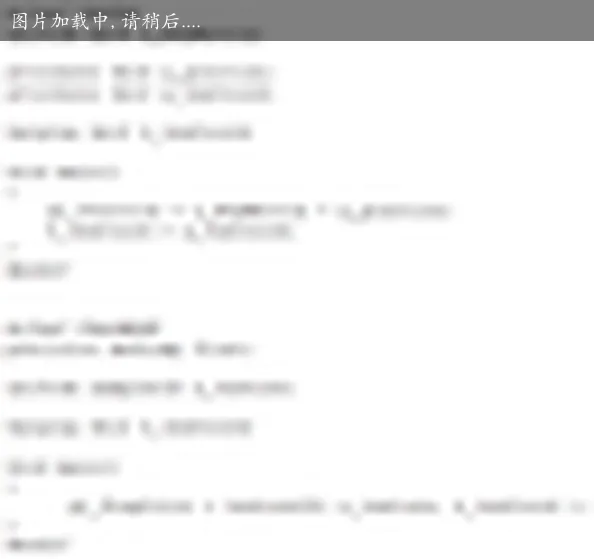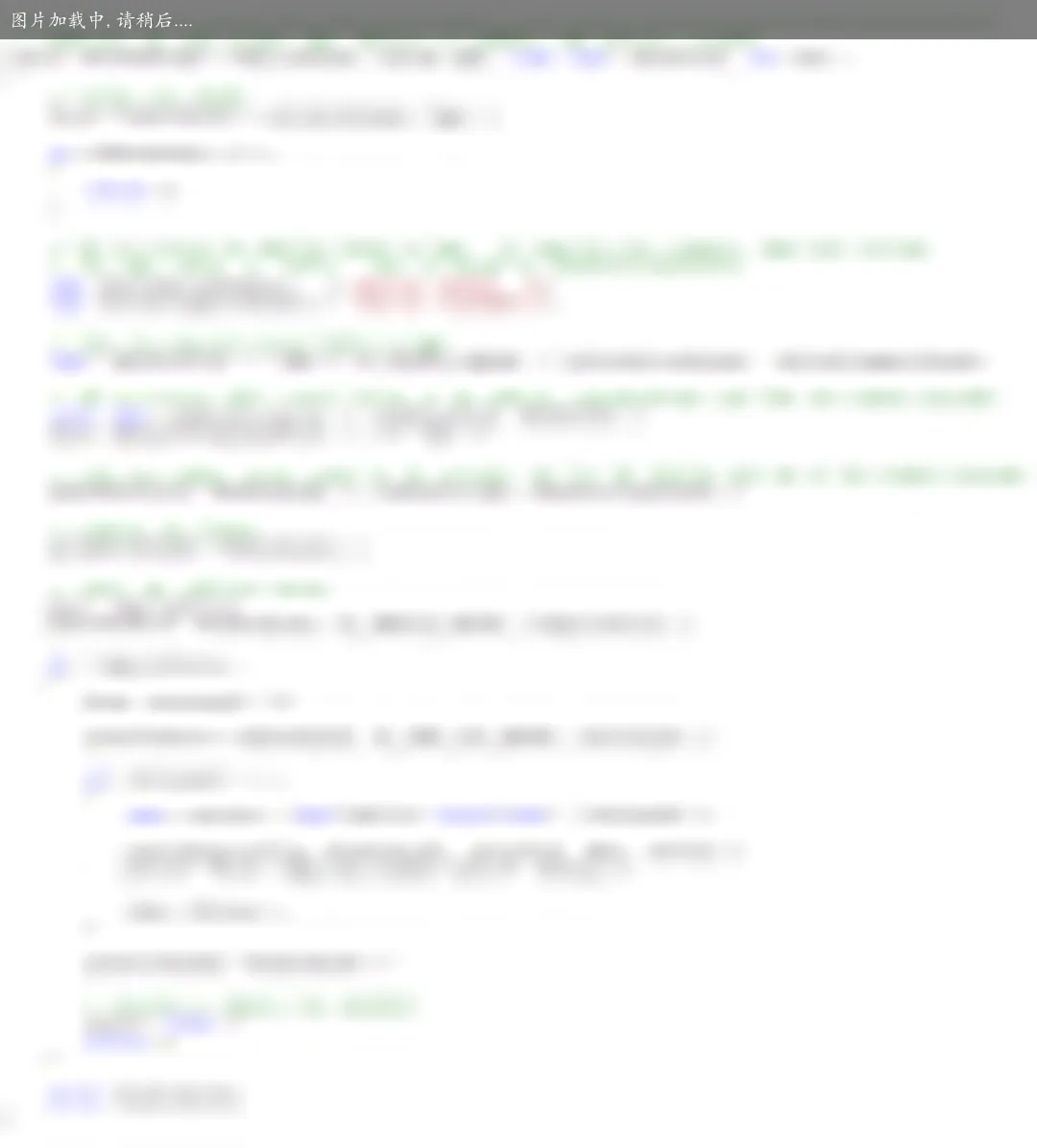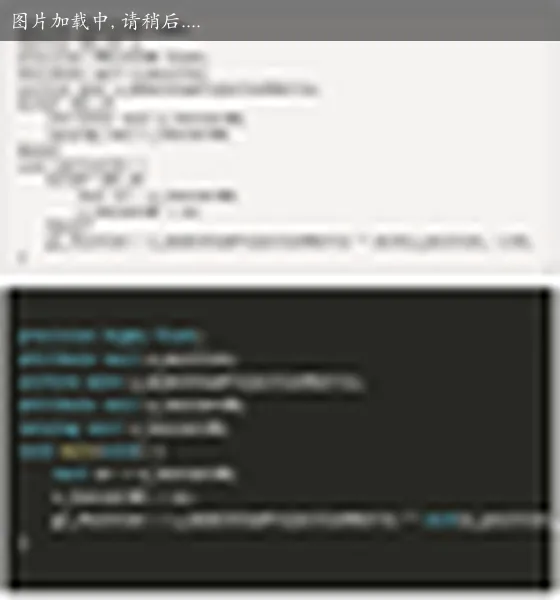If you have any questions or feedback, please reach out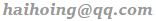.